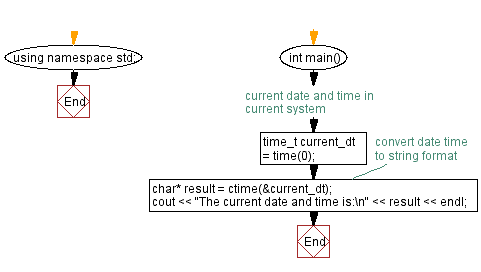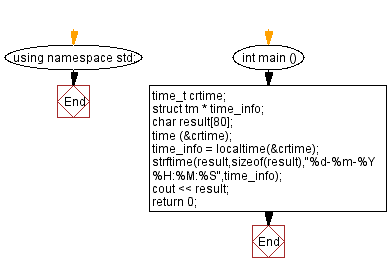﻿ C++ Exercises: Current date and time - w3resource

# C++ Exercises: Current date and time

## C++ Date: Exercise-1 with Solution

Write a C++ program to get the current date and time.

Sample Solution-1:

C++ Code:

``````#include <iostream>
#include <ctime>
using namespace std;

int main()
{
// current date and time in current system
time_t current_dt = time(0);

// convert date time to string format
char* result = ctime(&current_dt);

cout << "The current date and time is:\n" << result << endl;
}
``````

Sample Output:

```The current date and time is:
Tue Mar 15 14:28:05 2022
```

N.B.: The result may vary for your current system date and time.

Flowchart:Sample Solution-2:

C++ Code:

``````#include <iostream>
#include <ctime>
using namespace std;
int main ()
{
time_t crtime;
struct tm * time_info;
char result;
time (&crtime);
time_info = localtime(&crtime);
strftime(result,sizeof(result),"%d-%m-%Y %H:%M:%S",time_info);
cout << result;
return 0;
}

``````

Sample Output:

```15-03-2022 12:15:31
```

N.B.: The result may vary for your current system date and time.

Flowchart:C++ Code Editor: